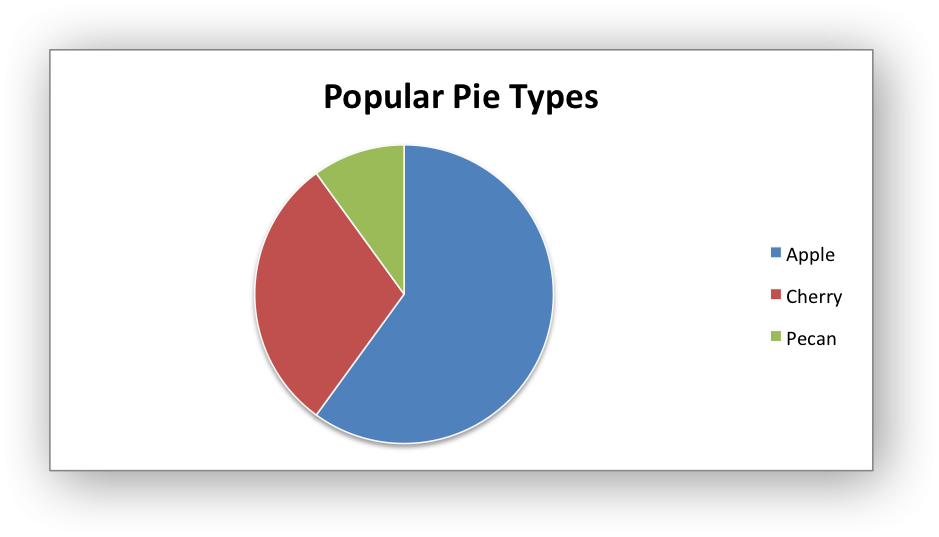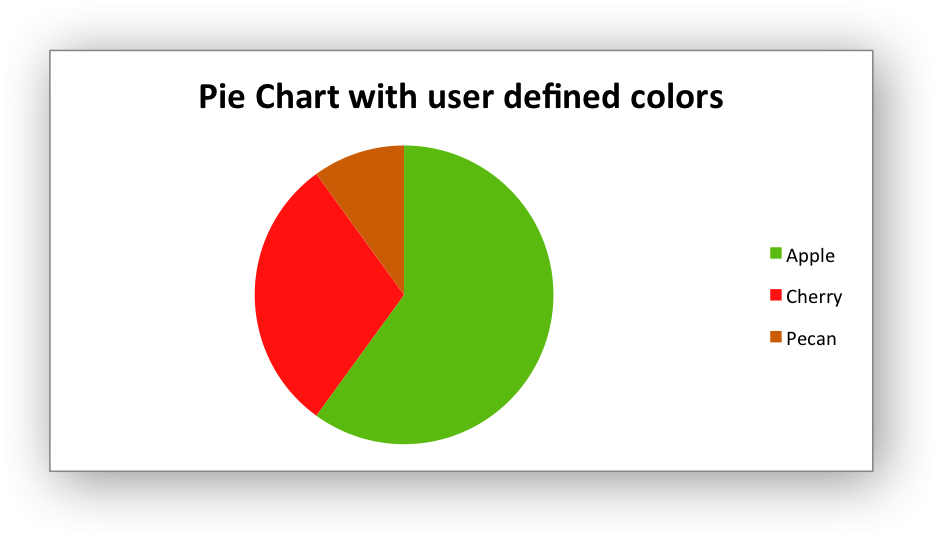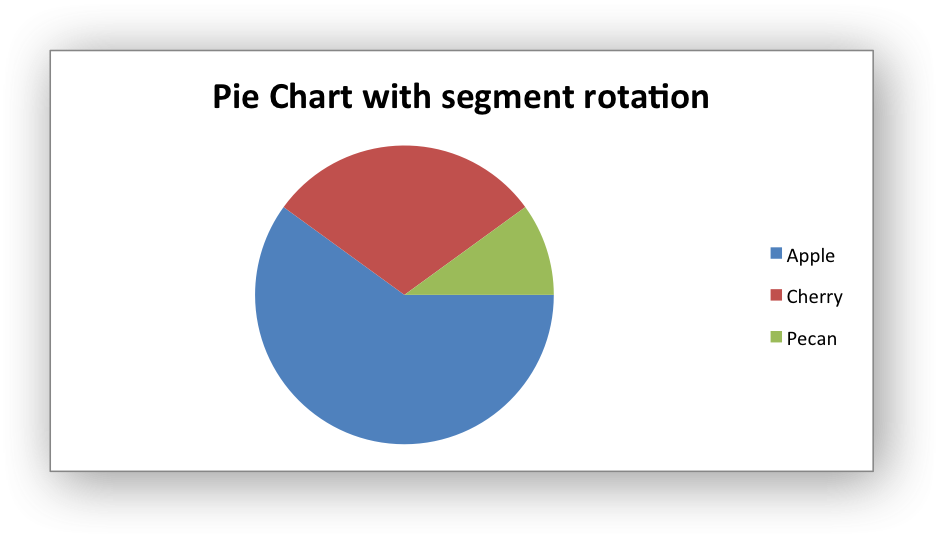libxlsxwriter
chart_pie.c

Examples of creating an Excel Pie chart.

The default pie chart:A pie chart with user defined colors:A pie chart with rotated segments:/*
* An example of creating an Excel pie chart using the libxlsxwriter library.
*
* The demo also shows how to set segment colors. It is possible to define
* chart colors for most types of libxlsxwriter charts via the series
* formatting functions. However, Pie/Doughnut charts are a special case since
* each segment is represented as a point so it is necessary to assign
* formatting to each point in the series.
*
* Copyright 2014-2021, John McNamara, jmcnamara@cpan.org
*
*/
#include "xlsxwriter.h"
/*
* Write some data to the worksheet.
*/
void write_worksheet_data(lxw_worksheet *worksheet, lxw_format *bold) {
worksheet_write_string(worksheet, CELL("A1"), "Category", bold);
worksheet_write_string(worksheet, CELL("A2"), "Apple", NULL);
worksheet_write_string(worksheet, CELL("A3"), "Cherry", NULL);
worksheet_write_string(worksheet, CELL("A4"), "Pecan", NULL);
worksheet_write_string(worksheet, CELL("B1"), "Values", bold);
worksheet_write_number(worksheet, CELL("B2"), 60, NULL);
worksheet_write_number(worksheet, CELL("B3"), 30, NULL);
worksheet_write_number(worksheet, CELL("B4"), 10, NULL);
}
/*
* Create a worksheet with examples charts.
*
*/
int main() {
lxw_workbook *workbook = workbook_new("chart_pie.xlsx");
lxw_chart *chart ;
/* Add a bold format to use to highlight the header cells. */
/* Write some data for the chart. */
write_worksheet_data(worksheet, bold);
/*
* Chart 1: Create a simple pie chart.
*/
/* Add the first series to the chart. */
/* Set the name for the series instead of the default "Series 1". */
chart_series_set_name(series, "Pie sales data");
/* Add a chart title. */
chart_title_set_name(chart, "Popular Pie Types");
/* Set an Excel chart style. */
chart_set_style(chart, 10);
/* Insert the chart into the worksheet. */
worksheet_insert_chart(worksheet, CELL("D2"), chart);
/*
* Chart 2: Create a pie chart with user defined segment colors.
*/
/* Add the first series to the chart. */
/* Set the name for the series instead of the default "Series 1". */
chart_series_set_name(series, "Pie sales data");
/* Add a chart title. */
chart_title_set_name(chart, "Pie Chart with user defined colors");
/* Add for fills for use in the chart. */
lxw_chart_fill fill1 = {.color = 0x5ABA10};
lxw_chart_fill fill2 = {.color = 0xFE110E};
lxw_chart_fill fill3 = {.color = 0xCA5C05};
/* Add some points with the above fills. */
lxw_chart_point point1 = {.fill = &fill1};
lxw_chart_point point2 = {.fill = &fill2};
lxw_chart_point point3 = {.fill = &fill3};
/* Create an array of the point objects. */
lxw_chart_point *points[] = {&point1,
&point2,
&point3,
NULL};
/* Add/override the points/segments of the chart. */
chart_series_set_points(series, points);
/* Insert the chart into the worksheet. */
worksheet_insert_chart(worksheet, CELL("D18"), chart);
/*
* Chart 3: Create a pie chart with rotation of the segments.
*/
/* Add the first series to the chart. */
/* Set the name for the series instead of the default "Series 1". */
chart_series_set_name(series, "Pie sales data");
/* Add a chart title. */
chart_title_set_name(chart, "Pie Chart with segment rotation");
/* Change the angle/rotation of the first segment. */
chart_set_rotation(chart, 90);
/* Insert the chart into the worksheet. */
worksheet_insert_chart(worksheet, CELL("D34"), chart);
return workbook_close(workbook);
}
chart_set_style
void chart_set_style(lxw_chart *chart, uint8_t style_id)
Set the chart style type.
workbook_close
lxw_error workbook_close(lxw_workbook *workbook)
Close the Workbook object and write the XLSX file.
chart_series_set_points
lxw_error chart_series_set_points(lxw_chart_series *series, lxw_chart_point *points[])
Set the formatting for points in the series.
chart_series_set_name
void chart_series_set_name(lxw_chart_series *series, const char *name)
Set the name of a chart series range.
LXW_CHART_PIE
@ LXW_CHART_PIE
Definition: chart.h:134
lxw_chart_series * chart_add_series(lxw_chart *chart, const char *categories, const char *values)
Add a data series to a chart.
workbook_new
lxw_workbook * workbook_new(const char *filename)
Create a new workbook object.
format_set_bold
void format_set_bold(lxw_format *format)
Turn on bold for the format font.
lxw_worksheet
Struct to represent an Excel worksheet.
Definition: worksheet.h:2107
lxw_format
Struct to represent the formatting properties of an Excel format.
Definition: format.h:358
lxw_chart_point::fill
lxw_chart_fill * fill
Definition: chart.h:786
lxw_chart
Struct to represent an Excel chart.
Definition: chart.h:1090
worksheet_insert_chart
lxw_error worksheet_insert_chart(lxw_worksheet *worksheet, lxw_row_t row, lxw_col_t col, lxw_chart *chart)
Insert a chart object into a worksheet.
lxw_chart_series
Struct to represent an Excel chart data series.
Definition: chart.h:960
chart_set_rotation
void chart_set_rotation(lxw_chart *chart, uint16_t rotation)
Set the Pie/Doughnut chart rotation.
lxw_chart_fill::color
lxw_color_t color
Definition: chart.h:666
lxw_chart_fill
Struct to represent a chart fill.
Definition: chart.h:663
lxw_workbook
Struct to represent an Excel workbook.
Definition: workbook.h:292
chart_title_set_name
void chart_title_set_name(lxw_chart *chart, const char *name)
Set the title of the chart.
worksheet_write_string
lxw_error worksheet_write_string(lxw_worksheet *worksheet, lxw_row_t row, lxw_col_t col, const char *string, lxw_format *format)
Write a string to a worksheet cell.
worksheet_write_number
lxw_error worksheet_write_number(lxw_worksheet *worksheet, lxw_row_t row, lxw_col_t col, double number, lxw_format *format)
Write a number to a worksheet cell.
lxw_chart_point
Struct to represent an Excel chart data point.
Definition: chart.h:780
CELL
#define CELL(cell)
Convert an Excel A1 cell string into a (row, col) pair.
Definition: utility.h:45